## Show that the kinetic energy of a particle of mass m is related to the magnitude of the momentum p of that particle by KE 5 p2/2m. (Note: Th

Question

Show that the kinetic energy of a particle of mass m is related to the magnitude of the momentum p of that particle by KE 5 p2/2m. (Note: This expression is invalid for particles traveling at speeds near that of light.)

in progress 0
4 months 2021-09-04T20:29:13+00:00 1 Answers 33 views 0

Kinetic energy: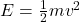Momentum: p = mv

Kinetic energy in terms of momentum: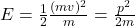Explanation:

The kinetic energy is given by this equation:(1)

Now, we know that the momentum of a particle is p = m*v. This equation is true only with a classical particle, it meas particles with a speed less than the speed of light. If we had a particle traveling at speeds near that of light, the momentum would be p = γm₀v, where γ is the Lorentz factor.

So, if we see, we can rewrite the equation (1) to get this expression in terms of p.

Let’s multiply and divide by mass (m) in the equation (1).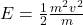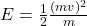Using the p = mv here: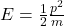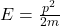Therefore the kinetic energy can express in terms of momentum.

Let’s see that it could not be possible using the the relativistic momentum, because it has a relativistic factor.

I hope it helps you!